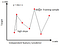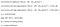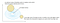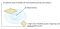# Day 42(ML & DL) — Lasso(L1) & Ridge(L2) Regularization Techniques for high variancePic from shopify

High variance is one of the most critical problems that need greater attention in ML & DL. The main reason for its occurrence is the lack of training data. The training set is considered to be complete when it strikes a balance between the number of features and the count of training instances(a.k.a) breadth Vs depth. With such dataset, the model will have a good understanding of the data patterns which in turn amplifies the prediction power of the model.

In contrast, a training dataset with sparsely populated samples will result in overfitting or high variance. Since the instances do not depict all the possible combinations of the attributes, a model with a jagged surface(with many spikes/peaks and valleys)is formed rather than a generalized smooth one. In real life, it is not always plausible to create an absolute training set. To overcome this limitation and design a powerful model, the regularization method is introduced.

Table of contents:

• Regularization for overfitting
• Ridge or L2 regularization
• Lasso or L1 regularization

What is regularization and how it helps to alleviate overfitting? A machine learning model with high variance and scattered data would end up having larger weighted coefficients(i.e) huge slopes. As per the equation of the straight line y = mx+ c (m corresponds to the slope), for a small change in x, there will be a proportionally larger change in y.Fig 1 — shows a model with high variance and overfitting

By reducing the slopes to make the model containing smooth surface prevents it from overfitting and also allows the model to produce better results for the test dataset.The formulas for different types of regularization techniques

Here the λ is a penalty term which prevents β from taking larger values. As the β is under control, the model will not have the sharp points rather it exhibits a smooth surface. Higher the value of λ more penalty is issued so the coefficients are drastically reduced and sometimes results in underfitting. Lower the value of λ less penalty is issued and the model may still be high variance. So an optimum value of λ should be given.

Ridge Regularization or L2: The regularization parameter is quadratic(beta squared). As a result, the regularization term results in a circle. So, the idea here is to find the global minima under the constraint of the regularization term. This implies that the minimum cost is found at the intersection of both the terms in the loss function. This is same as the LaGrange multiplier optimization problem.Fig2 — shows ridge regularization with optimum & high values of lambda

low lambda — The circle will be huge and it will touch exactly the global minima where the coefficients beta is higher. This is the case of high variance.

medium lambda — It is the resultant of the regularization, where the variance has been reduced and the model is expected to perform equally in both test and train.

high lambda — It shrinks the circle and the convergence happens at small coefficients and the model posses extreme smooth surface which makes it underfit with high bias.

Lasso Regularization or L1: Most of the above-said points are applicable to Lasso similar to the ridge. The main differentiating factor between the two methods is that, the shape of the constraint. In the case of the ridge, the constraint forms a circle and the intersection points have non-zero coordinate values. As a result, some of the dimensions have very tiny value but not completely downsized to zero. On the other hand, if we take Lasso the constraints take the shape of the square or flat surface which allows it to touch the optimal minimum cost values with some dimensions equal to zero.

This salient feature of Lasso regularization method helps to reduce the dimensions as some of the coefficients would become zero implicitly conveying they do not play a significant role as predictors.Fig 3 — shows Lasso Regularization

Regularization and Feature selection for Regression: https://github.com/NandhiniN85/Regularization-Technique/blob/master/Ridge%20and%20Lasso%20regression.ipynb

Regularization and Feature Selection for Classification: https://github.com/NandhiniN85/Regularization-Technique/blob/master/Lasso%20classification.ipynb

Recommended Reading:

AI Enthusiast | Blogger✍

## More from Nandhini N

AI Enthusiast | Blogger✍